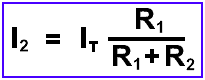# Current Divider Rule Calculator – CDR Formula & Calculations

## CDR – Current Divider Rule Calculator for Current Division

### Current Divider Rule

Current diver rule aka CDR or current division is a basic technique used to determine the value of currents flowing in the electrical elements such as inductors, capacitors or resistors connected in parallel combination. On the other hand, the Voltage divider rule “VDR ” is used to find the flowing current in the elements connected in series circuits.

This is because current is the same in the parallel circuit while voltage is different. Similarly, voltage is the same in parallel circuits while current is different. Keep in mind that current always chooses the least resistances and impedances path to flow in it.Related Posts:

### Current Divider Rule Calculator

The following Current Divider Calculator can be used to calculate for current division having multiple elements in parallel combination.

Enter three values (Input current, and both resistances) and click on calculate button. The resultant current in the resistors will be displayed in the result box.

 IT AmAMΩµA R1 ΩkΩMΩGΩ R2 ΩkΩMΩGΩ I1 AmAMΩµA I2 AmAMΩµA Round to Decimal ###.##.###.####.#####.######

Related Posts:

### Formula for CDR Calculator

The following basic formulas can be used for DC circuits using current divider rule.

In = IT x  (Rn / (R1 + R2))

Current in R1Current in R2Where

• IT = Total Input Current
• R1+ R2 = Resistors values
• I1 , I2 = Desired value of currents in the resistors

Similarly, the following formulas can be used for AC circuits using CDR.

In = IT x  (Zn / (Z1 + Z2))

Where Z is the impedances (resistances (like inductive and capacitive reactances “XL & XC” in AC circuits).

### Example (To Verify the CDR):

Find the current passes through both resistors using current divider rule for the given circuit.To solve the circuit, we will have to first find the equivalent resistance and total input current.

Equivalent Resistance:

1 / Req = 1 / R1 + 1 / R2

1 / Req = 1 / 60 + 1 / 20

Req = 15 Ω

Total input current supplied by 120V Voltage source (using Ohm’s Law):

IT = V / R

IT = 120 / 15

IT = 8 A

Now, we can find the value of currents flowing through both R1 and R2 resistors using CDR:

Current I1 in Resistor 1.

I1 = IT x  (R2 / (R1 + R2))

I1 = 8A x  (20Ω / (60Ω + 20Ω))

I1 = 2A

Current I2 in Resistor 2:

I2 = IT x  (R1 / (R1 + R2))

I2 = 8A x  (60Ω / (20Ω + 60Ω))

I2 = 6A

You may verify the answers using the CDR calculator given above.

Related Calculators: